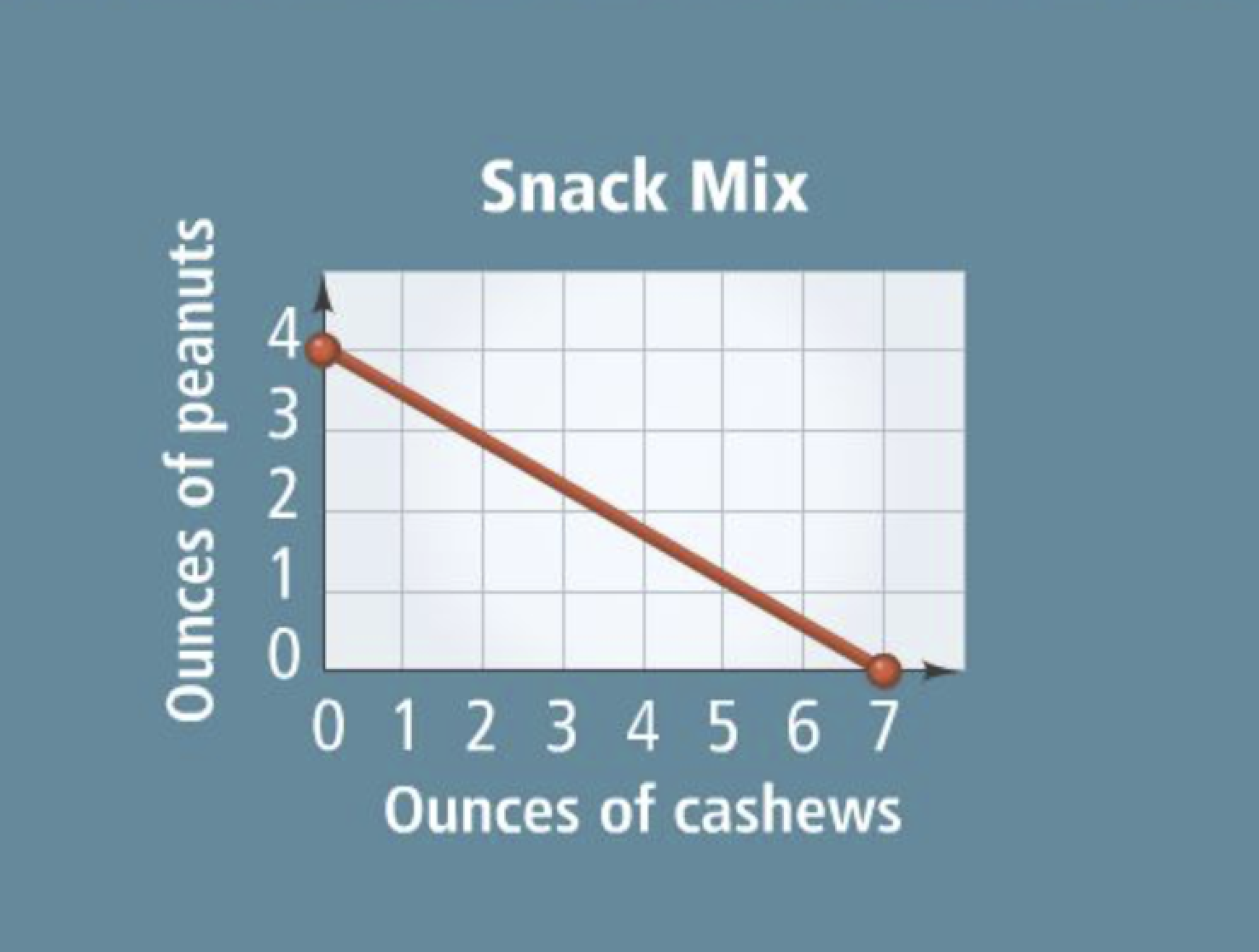Algebra 1 5-5 Guided Practice: Standard Form
starstarstarstarstarstarstarstarstarstar
by Matthew Richardson
| 17 Questions1
2
1
10
Solve It! An athlete wants to make a snack of peanuts and cashews that will contain a certain amount of protein. Cashews have 4 g of protein per ounce and peanutes have 7 g of protein per ounce. Based on the graph, how many grams of protein will the athlete's mix contain?
36
28
0
14
2
10
Solve It! What do the points (7, 0) and (0, 4) represent in the context of the scenario?a
3
4
5
6
Recall:
General Slope-intercept form equation:
General Point-slope form equation:

3
10
Take Note: Write the general standard form equation. (Capitalization matters)
4
10
Take Note: What is a y-intercept ?
5
10
Take Note: What is an x-intercept ?
6
10
Take Note: How can you find the x-intercept of an equation written in standard form ?7
7
10
Problem 1 Got It?
A
B
C
D8
8
10
Problem 1 Got It?
A
B
C
D
9
10
Problem 2 Got It? What is the graph of the equation?
Be sure to include relevant graph detail: label axes, indicate units on both axes, and use arrows to represent end behavior, as appropriate.
10
10
Problem 3 Got It? What is the graph of the equation?
Be sure to include relevant graph detail: label axes, indicate units on both axes, and use arrows to represent end behavior, as appropriate.
11
10
Problem 3 Got It? What is the graph of the equation?
Be sure to include relevant graph detail: label axes, indicate units on both axes, and use arrows to represent end behavior, as appropriate.
12
10
Problem 3 Got It? What is the graph of the equation?
Be sure to include relevant graph detail: label axes, indicate units on both axes, and use arrows to represent end behavior, as appropriate.
13
10
Problem 3 Got It? What is the graph of the equation?
Be sure to include relevant graph detail: label axes, indicate units on both axes, and use arrows to represent end behavior, as appropriate.14
14
10
Problem 4 Got It?
A
B
C
D15
16
15
10
Problem 5 Got It? In Problem 5, suppose the store charged \$15 for each movie. What equation describes the numbers of songs and movies you can purchase for \$60?
x + 15y = 60
15x + 12y = 60
15x + y = 60
16
10
Problem 5 Got It? Reasoning: What domain and range are reasonable for the equation in the previous item? Explain.17
17
10
Take Note: Summarize the content of this lesson.